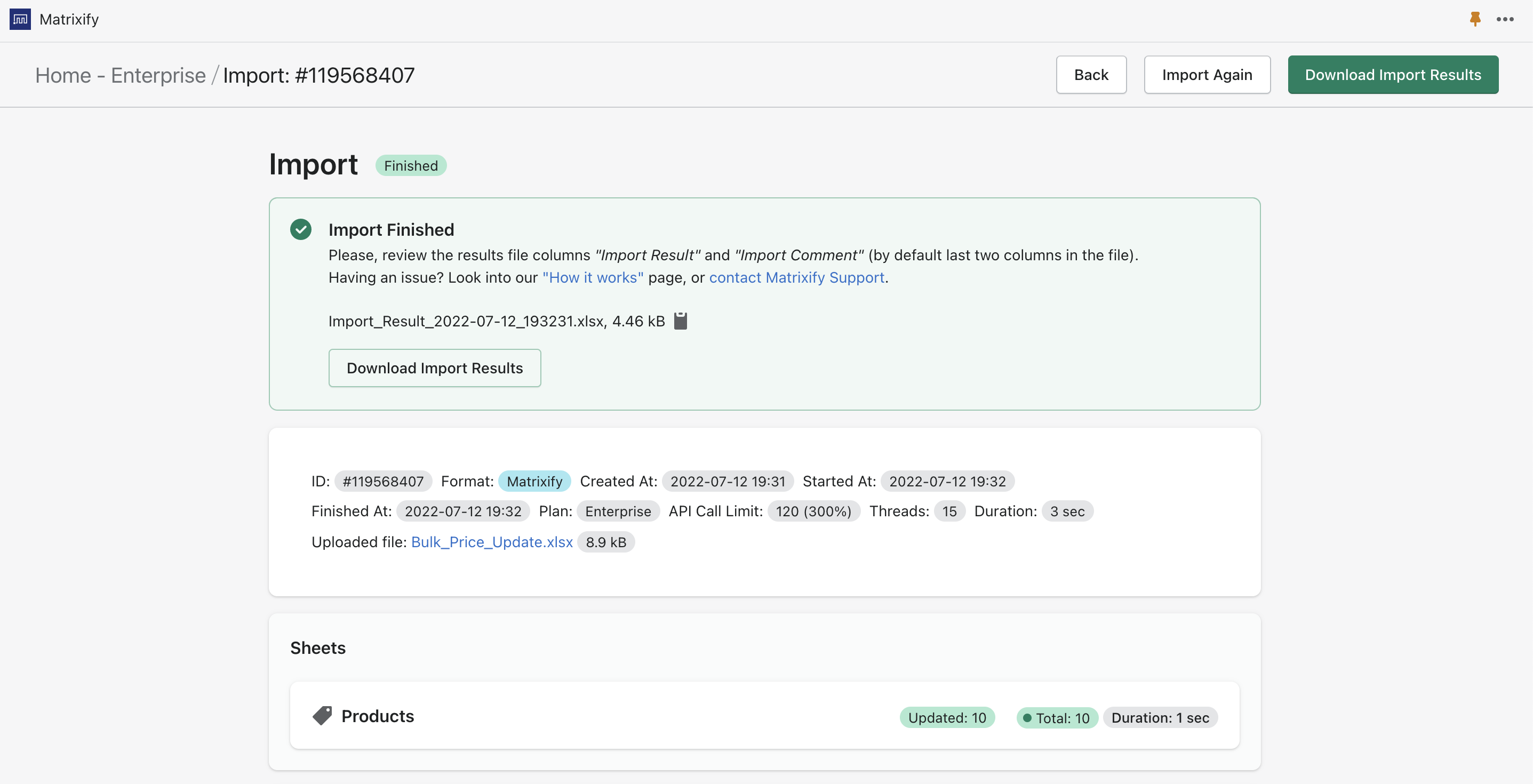# How to add specific amount to your Shopify Product price in bulkLooking for an easy way to add a few dollars or few percents to your Shopify Products? Let’s take a look at how to do it with the Matrixify.

## 1. Export Products

In the Matrixify apps Export section, select the checkbox Products from the “Select Sheets” drop-down. The select Basic Columns and Inventory / Variants.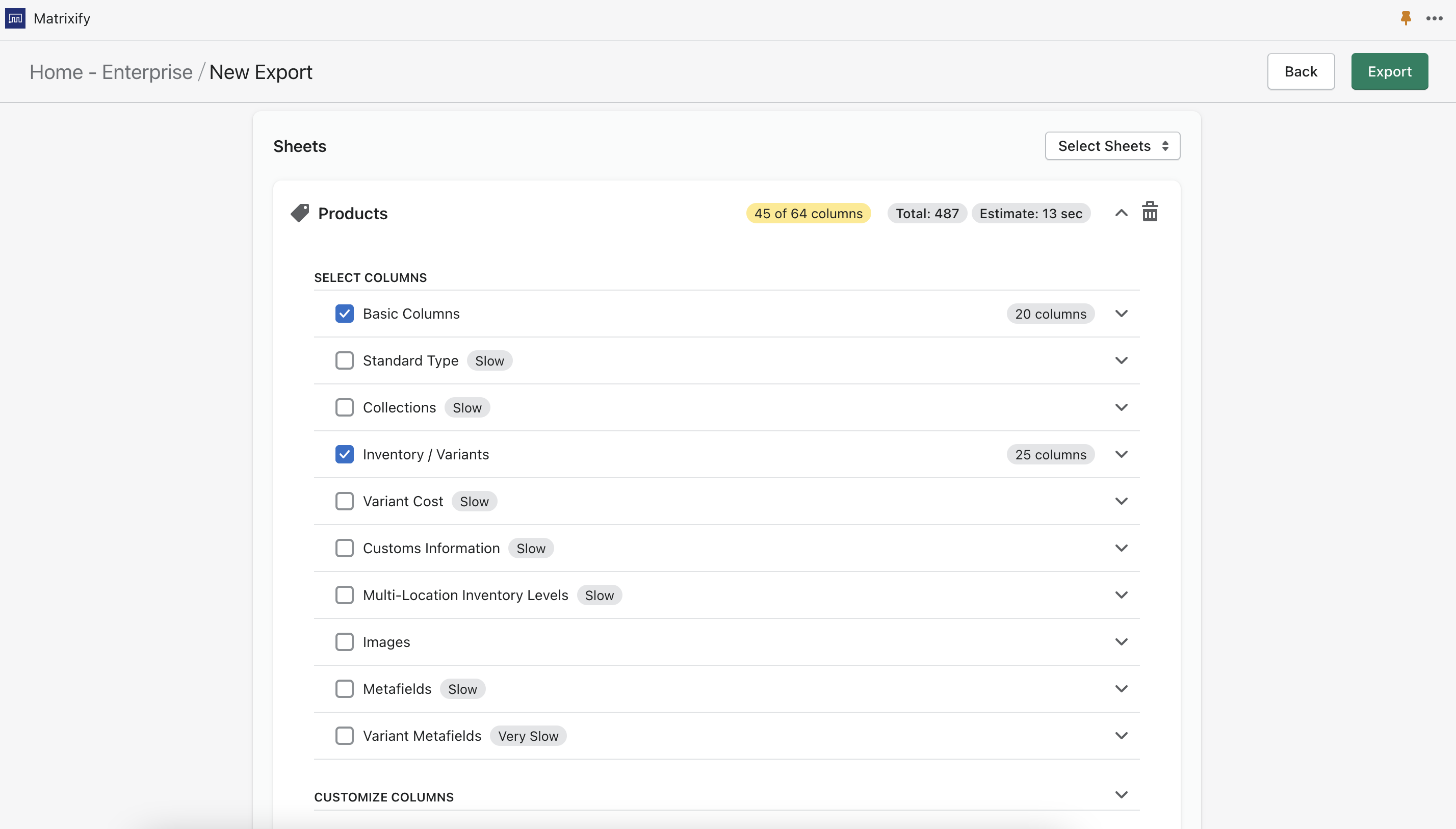You can also apply filters to limit the Products you are exporting, for example, to export only the Products that are in specific Collection.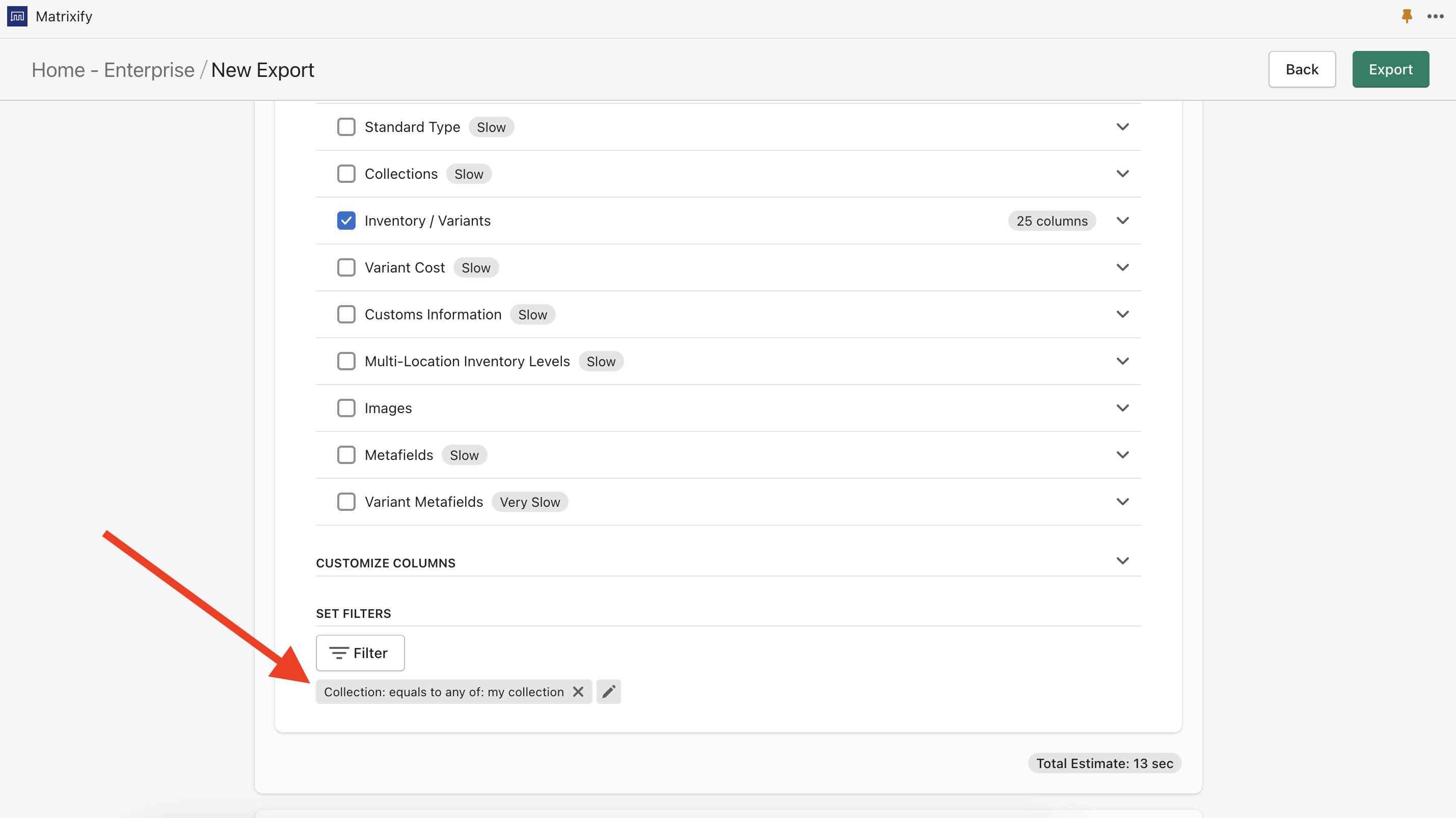Select format Matrixify: Excel and press the Export button to start the export.

## 2. Delete unnecessary columns

Since we do not wish to update any other fields than Variant Price, then we do not need to include all the columns in the file.
You will only need the following columns:

## 3. Rename Variant Price column and add a new one

The Matrixify app will ignore all columns it does not recognize, so you can rename the current Variant Price column to anything that is not recognized by the app, so you can use it in formulas for new Variant Price.

It helps to always just add the word “Ignore” to the column name since no columns in our template contain this word.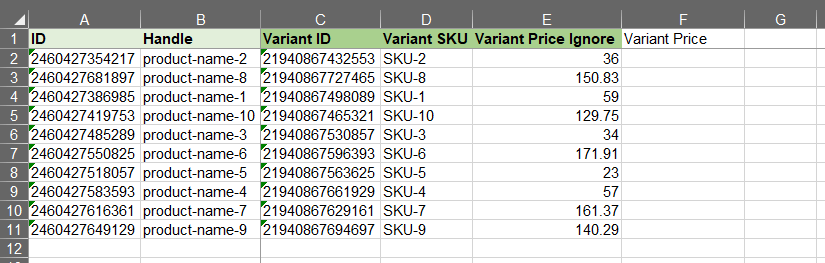## 4. Construct the formula

For example, let’s take a look at how to add a specific amount and how to add specific percent.

To add a specific amount, in my case 15, use formula the following in cell F2.

`=E2+15`

This formula will take the value from cell E2 and add 15 to it.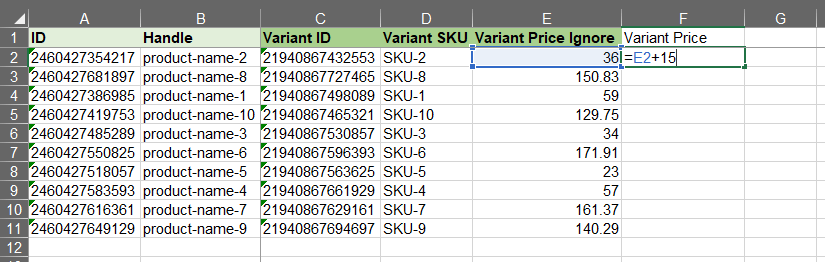### Add percent to the amount:

To add a percent, in my case 10%, use formula the following formula in cell F2

`=E2+E2*10%`

Let’s break down this formula:

=E2+E2*10%

E2 = value in cell E2
E2*10% = 10 percent from the value in cell E2

This formula thus will take the value from the cell E2, then calculate 10 percent from E2 and add both together, returning the value in cell F2 where the formula is located.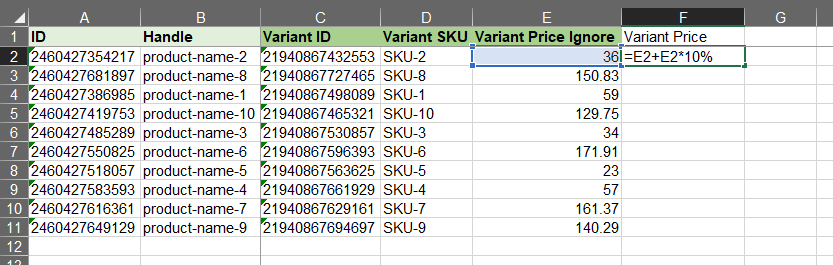### Apply the formula to the whole column

Select cell F2 and double click on the square in the bottom-right corner to apply this formula to the whole column.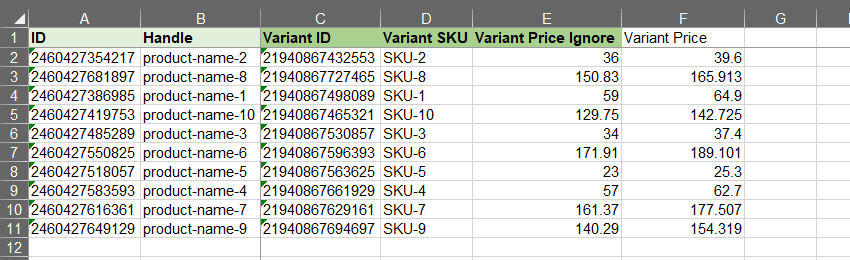### Other noteworthy formulas

=ROUND(number, num_digits)

Rounds a number to a specified number of digits.

=ROUNDUP (number, num_digits)

Returns a number rounded up to a given number of decimal places. Unlike standard rounding, where numbers less than 5 are rounded down, ROUNDUP always rounds numbers 1-9 up.

=CEILING (number, multiple)

Returns a given number rounded up to a specified multiple. For example, =CEILING(E2,5) could be used to round a price in E2 to the nearest 5 dollars. CEILING always rounds up.

## 5. Save, import and enjoy.

Now you can save the file. Go back to the Matrixify app and import the file in the Import section. The app will analyze the file and count how many Products you are importing.

Press the Import button to start the import.

If you see any Failed items, then after the import has finished, you can download the Import Results file and look into the “Import Comment” column. It will tell why particular Products has failed. Then you can fix that issue and re-import just those failed and fixed rows again.